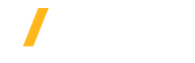# Ansys Parametric Licensing Calculator

Use the form below to calculate the amount of HPC licensing needed to run concurrent parametric analyses with many Ansys solvers (such as Ansys Mechanical, Fluent, HFSS, etc.). Simply input the number of concurrent parametric analyses you wish to solve and how many cores you would like each analysis to run on.

For example, six concurrent analyses on eight cores each.

Only non zero, non negative integers and max 1,000,000 can be entered for the number of analyses and cores